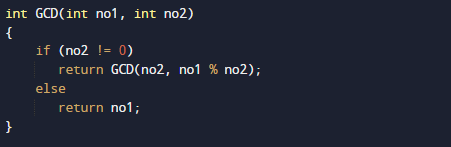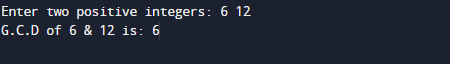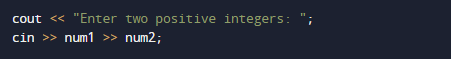# C++ Program to Find GCD Using Recursion

In this tutorial you will learn about the C++ Program to Find GCD Using Recursion and its application with practical example.

In this tutorial, we will learn to create a c++ program that will find GCD using recursion in c++ programming.

Prerequisites

Before starting with this tutorial we assume that you are best aware of the following C++ programming topics:

• Operators.
• If-else statements.
• Basic input/output.
• Basic c++ programming
• Recursion.
• Nested if else statement.
• User Define Functions.

## What Is  Recursion?

Recursion is a process of repeating itself in a similar way. if a function call itself inside the same function, then it is called a recursive calling of a function.
Recursion means a  function is called itself the same function, it is known as recursion in C++.## What Is GCD?

GCD(Greatest Common Divisor) or HCF (Highest Common Factor) . When  we finding the factor of two numbers, Highest Common Factor of  numbers is the Largest factor that divides these two numbers. The greatest factor found from the common factors is called the HCF. It is also called the Greatest common factor.

## C++ Program to Find GCD Using Recursion.

In this program we will find GCD of two given number using Recursion . We would first declared and initialized the required variables. Next, we would prompt user to input two numbers.Later we will find the GCD of given number using Recursion and user define function.

## OutputIn the above program, we have first declared and initialized a set variables required in the program.

• num1 =It will hold first number given by user.
• num2 =It will hold second number given by user.
• no1= It will hold first number given by user in function.
• no2=It will hold second number given by user in function.

Let see the step by step method of finding GCD.

GCD of two number is :
1: user will be prompted to enter two the positive integer values num1 and num2.
2: Storing the given value in variable  num1 & num2  as shown below.3:Now pass these two value to function GCD(num1,num2) .Where we will calculate GCD using recursion method .As we can see in above image function GCD calling itself until it satisfied the condition and returns the Greatest common Divisor of two numbers.

In this tutorial we have learn about the C++ Program to Find GCD Using Recursion and its application with practical example. I hope you will like this tutorial.